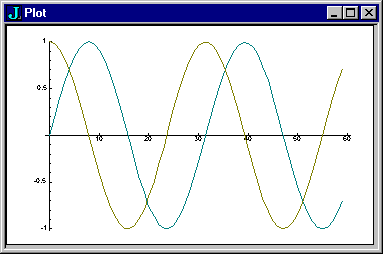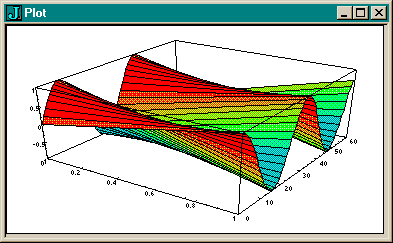>>  <<  Usr  Pri  JfC  LJ  Phr  Dic  Rel  Voc  !:  Help  Primer

The dyad v/ computes a table for the verb v (a function table).
```   a =. i. 5
a +/ a	NB. addition table
0 1 2 3 4
1 2 3 4 5
2 3 4 5 6
3 4 5 6 7
4 5 6 7 8
a */ a	NB. times table
0 0 0  0  0
0 1 2  3  4
0 2 4  6  8
0 3 6  9 12
0 4 8 12 16
0 1 +/ 0 1	NB. + table on booleans
0 1
1 2
0 1 +./ 0 1	NB. or
0 1
1 1
0 1 +:/ 0 1	NB. not-or
1 0
0 0
0 1 */ 0 1	NB. times
0 0
0 1
0 1 *./ 0 1	NB. and
0 0
0 1
0 1 *:/ 0 1	NB. not-and
1 1
1 0```
The derived verb v/ has a left rank that is the left rank of v and a right rank of _ ; it applies v between each cell of the left argument and the entire right argument.
`   additiontable =. +/`
The verb additiontable is defined as the result of the / adverb applied to + as an argument. The definition is the same as the definition for sumover that you used in the previous section. The name additiontable makes sense when the dyad is used, and sumover makes sense when the monad is used. Nothing prevents using either name in a misleading way.
```   a additiontable a
0 1 2 3 4
1 2 3 4 5
2 3 4 5 6
3 4 5 6 7
4 5 6 7 8```
The left rank of + is 0, so the rank of the derived verb is 0 and its cell arguments are atoms. The right rank of the derived verb is _ and its cell arguments are the entire right argument. Visually the above works as follows:
```0 (first atom from left) + entire right   gives  0 1 2 3 4
1 (next atom from left)  + entire right   gives  1 2 3 4 5
2 (next atom from left)  + entire right   gives  2 3 4 5 6
3 (next atom from left)  + entire right   gives  3 4 5 6 7
4 (next atom from left)  + entire right   gives  4 5 6 7 9```
The following is an interesting use of table together with the plotting from an earlier section. The plot facility plots each row in a table argument as a separate series of data.
```   load 'plot'
plot 1 2 o. / 0.2 * i.60   ``````	'surface' plot 1 2 o. / 0.2 * i.60
```The 1 from the left argument used as the left argument of o. gives sine values in the first row. The 2 from the left argument for o. gives cosine values in the second row.

In the examples above the left rank of the original verb is always 0 and so the cells of the left argument of the derived verb are simply the atoms of the left argument. Let's look at an example where the left rank of the original verb is not 0.
`   f =. ,"1`
The verb f is append with a left and right rank of 1.
```   p=. 2 2 \$'abcd'
p
ab
cd
q=.3 3\$'ABCD'
q
ABC
DAB
CDA

p f/ q
abABC
abDAB
abCDA

cdABC
cdDAB
cdCDA```
Visually:
```ab (1st list from left) , entire right   gives	abABC
abDAB
abCDA

cd (next list)    	, entire right   gives	cdABC
cdDAB
cdCDA
```

>>  <<  Usr  Pri  JfC  LJ  Phr  Dic  Rel  Voc  !:  Help  Primer